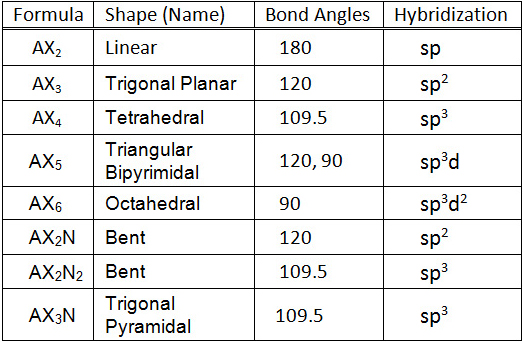# What mode of hybridization is associated with each of the five common electron domain geometries?

Jun 6, 2015

The hybridization uses first $s$ orbitals, then $p$ orbitals, and finally $d$ orbitals.

We can classify the electron geometries according to the ${\text{AX}}_{n}$ system, and the total number of orbitals used is equal to $n$.

• ${\text{AX}}_{2}$ = linear = $s p$ hybridization
• ${\text{AX}}_{3}$ = trigonal planar = $s {p}^{2}$ hybridization
• ${\text{AX}}_{4}$ = tetrahedral = $s {p}^{3}$ hybridization
• ${\text{AX}}_{5}$ = trigonal bipyramidal = $s {p}^{3} d$ hybridization
• ${\text{AX}}_{6}$ = octahedral = $s {p}^{3} {d}^{2}$ hybridization